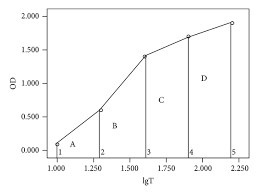## How to Calculate and Solve for Total Mass Determination | GravityThe image above represents total mass determination.

To compute for total mass determination, two essential parameters are needed and these parameters are Maximum Gravity (Δgmaxand Half Width (x1/2).

The formula for calculating total mass determination:

M ≃ 255Δgmax(x1/2

Where:

M = Total Mass
Δgmax = Maximum Gravity
x1/2 = Half Width

Let’s solve an example;
Find the total mass determination when the maximum gravity is 10 and the half width is 6.

This implies that;

Δgmax = Maximum Gravity = 10
x1/2 = Half Width = 6

M ≃ 255Δgmax(x1/2
M ≃ 255(10)(6)²
M ≃ 255(10)(36)
M ≃ 91800

Therefore, the total mass determination is 91800 kg.